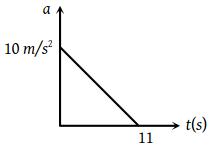If a freely falling body travels in the last second a distance equal to the distance travelled by it in the first three second, the time of the travel is

(1) 6 sec

(2) 5 sec

(3) 4 sec

(4) 3 sec

Concept Questions :-

Uniformly accelerated motion
High Yielding Test Series + Question Bank - NEET 2020

Difficulty Level:

The effective acceleration of a body, when thrown upwards with acceleration a will be :

(1) $\sqrt{a-{g}^{2}}$

(2) $\sqrt{{a}^{2}+{g}^{2}}$

(3) $\left(a-g\right)$

(4) $\left(a+g\right)$

Concept Questions :-

Uniformly accelerated motion
High Yielding Test Series + Question Bank - NEET 2020

Difficulty Level:

A body is thrown vertically upwards with velocity u. The distance travelled by it in the fifth and the sixth seconds are equal. The velocity u is given by (g = 9.8 m/s2

(1) 24.5 m/s

(2) 49.0 m/s

(3) 73.5 m/s

(4) 98.0 m/s

Concept Questions :-

Uniformly accelerated motion
High Yielding Test Series + Question Bank - NEET 2020

Difficulty Level:

A body, thrown upwards with some velocity reaches the maximum height of 50 m. Another body with double the mass thrown up with double the initial velocity will reach a maximum height of

(1) 100 m

(2) 200 m

(3) 300 m

(4) 400 m

Concept Questions :-

Uniformly accelerated motion
High Yielding Test Series + Question Bank - NEET 2020

Difficulty Level:

A parachutist after bailing out falls 50 m without friction. When parachute opens, it decelerates at 2 m/s2. He reaches the ground with a speed of 3 m/s. At what height, did he bail out ?

(1) 293 m

(2) 111 m

(3) 91 m

(4) 182 m

Concept Questions :-

Uniformly accelerated motion
High Yielding Test Series + Question Bank - NEET 2020

Difficulty Level:

When a ball is thrown up vertically with velocity V0, it reaches a maximum height of 'h'. If one wishes to triple the maximum height then the ball should be thrown with velocity

1. $\sqrt{3}{V}_{o}$

2. 3 V0

3. 9 V0

4. 3/2 V0

Concept Questions :-

Uniformly accelerated motion
High Yielding Test Series + Question Bank - NEET 2020

Difficulty Level:

A particle moving in a straight line covers half the distance with speed of 3 m/s. The other half of the distance is covered in two equal time intervals with speed of 4.5 m/s and 7.5 m/s respectively. The average speed of the particle during this motion is

(1) 4.0 m/s

(2) 5.0 m/s

(3) 5.5 m/s

(4) 4.8 m/s

Concept Questions :-

Average speed and average velocity
High Yielding Test Series + Question Bank - NEET 2020

Difficulty Level:

The acceleration of a particle is increasing linearly with time t as bt. The particle starts from the origin with an initial velocity v0 The distance travelled by the particle in time t will be

(1) ${v}_{0}t+\frac{1}{3}b{t}^{2}$

(2) ${v}_{0}t+\frac{1}{3}b{t}^{3}$

(3) ${v}_{0}t+\frac{1}{6}b{t}^{3}$

(4) ${v}_{0}t+\frac{1}{2}b{t}^{2}$

Concept Questions :-

Non-uniform acceleration
High Yielding Test Series + Question Bank - NEET 2020

Difficulty Level:

A particle starts from rest. Its acceleration (a) versus time (t) is as shown in the figure. The maximum speed of the particle will be1. 110 m/s

2. 55 m/s

3. 550 m/s

4. 660 m/s

Concept Questions :-

Graphs
High Yielding Test Series + Question Bank - NEET 2020

Difficulty Level:

A car accelerates from rest at a constant rate α for some time, after which it decelerates at a constant rate β and comes to rest. If the total time elapsed is t, then the maximum velocity acquired by the car is

(1) $\left(\frac{{\alpha }^{2}+{\beta }^{2}}{\alpha \beta }\right)\text{\hspace{0.17em}}t$

(2) $\left(\frac{{\alpha }^{2}-{\beta }^{2}}{\alpha \beta }\right) t$

(3) $\frac{\left(\alpha +\beta \right)\text{\hspace{0.17em}}t}{\alpha \beta }$

(4) $\frac{\alpha \beta \text{\hspace{0.17em}}t}{\alpha +\beta }$

Concept Questions :-

Acceleration